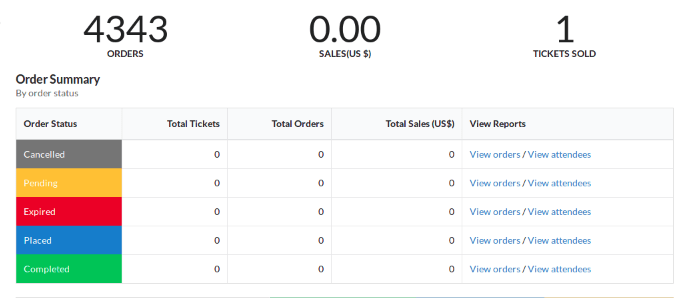# Implementing Order Statistics API on Tickets Route in Open Event Frontend

The order statistics API endpoints are used to display the statistics related to tickets, orders, and sales. It contains the details about the total number of orders, the total number of tickets sold and the amount of the sales. It also gives the detailed information about the pending, expired, placed and completed orders, tickets, and sales.

This article will illustrate how the order statistics can be displayed using the Order Statistics API in Open Event Frontend. The primary end point of Open Event API with which we are concerned with for statistics is

```GET /v1/events/{event_identifier}/order-statistics
```

First, we need to create a model for the order statistics, which will have the fields corresponding to the API, so we proceed with the ember CLI command:

```ember g model order-statistics-tickets
```

Next, we need to define the model according to the requirements. The model needs to extend the base model class. The code for the model looks like this:

```import attr from 'ember-data/attr';
import ModelBase from 'open-event-frontend/models/base';

export default ModelBase.extend({
orders  : attr(),
tickets : attr(),
sales   : attr()
});
```

As we need to display the statistics related to orders, tickets, and sales so we have their respective variables inside the model which will fetch and store the details from the API.

Now, after creating a model, we need to make an API call to get the details. This can be done using the following:

```return this.modelFor('events.view').query('orderStatistics', {});
```

Since the tickets route is nested inside the event.view route so, first we are getting the model for event.view route and then we’re querying order statistics from the model.

The complete code can be seen here.

Now, we need to call the model inside the template file to display the details. To fetch the total orders we can write like this

```{{model.orders.total}}
```

In a similar way, the total sales can be displayed like this.

```{{model.sales.total}}
```

And total tickets can be displayed like this

```{{model.tickets.total}}
```

If we want to fetch other details like the pending sales or completed orders then the only thing we need to replace is the total attribute. In place of total, we can add any other attribute depending on the requirement. The complete code of the template can be seen here.

The UI for the order statistics on the tickets route looks like this.Fig. 1: The user interface for displaying the statistics

The complete source code can be seen here.

Resources: